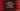# Python program to find out the sum of all digits of a number## Introduction :

In this python programming tutorial, we will learn how to find out the total sum of all digits of a number. For example, if the number is 123, the program will print 6 as the output. Our program will take the number as input from the user.

## Algorithm :

The algorithm that we are going to use is like below :

1. Ask the user to enter a number.

2. Read the number and store it in a variable.

3. Initialize one variable to zero for storing the sum.

4. Using a loop, get the last digit of the number. Add the digit to the ‘sum’ variable.

5. Remove the last digit from the number.

6. Keep adding the last digit to the ‘sum’ variable until the number becomes zero.

7. Print out the result to the user.

### Python program :

``````#1
num = int(input("Enter a number : "))
#2
original_num = num
#3
sum = 0

#4
while(num > 0):
#5
last_digit = num % 10
sum = last_digit + sum
num = num//10

#6
print("The sum of all digits of {} is {}".format(original_num,sum))
``````

### Explanation :

The commented numbers in the above program denote the step numbers below :

1. __Ask the user to enter a number. Read and store the number in variable num.

2. We are storing the same user input value in original_num variable as well. This variable will be used to print out the number to the user.

3. Create one variable sum with its value as ‘0’.

4. Run one while loop till the value of num is positive non zero.

5. First, get the last digit of the number using % operator. Add it to the sum variable and change the number as number/10

6. After the loop is completed, print out the sum of the digits to the user.

### Sample Output :

``````Enter a number : 387
The sum of all digits of 387 is 18

Enter a number : 222
The sum of all digits of 222 is 6

Enter a number : 123
The sum of all digits of 123 is 6

Enter a number : 566732
The sum of all digits of 566732 is 29
``````

### Conclusion :

In this tutorial, you have learned how to get the sum of all digits of a number. Using the same method, you can iterate through each digit and find out the multiplication of all numbers as well. This method is useful if you need to iterate through the digits. Try to run the program on your machine and drop one comment below if you have any queries.